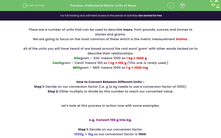# Understand Metric Units of Mass

In this worksheet, students will practise converting between four units of mass measurement (kilogram, centigram, milligram and gram) by finding a conversion factor and deciding whether to multiply or divide by this to find an equivalent amount.Key stage:  KS 4

Year:  GCSE

GCSE Subjects:   Maths

GCSE Boards:   AQA, Eduqas, Pearson Edexcel, OCR,

Popular topics:   Geometry worksheets

Difficulty level:#### Worksheet Overview

There are a number of units that can be used to describe mass, from pounds, ounces and tonnes to stones and grams.

We are going to focus on the most common of these which is the metric measurement grams.

All of the units you will have heard of are based around the root word 'gram' with other words tacked on to describe their relationships:

Kilogram - 'Kilo' means 1000 so 1 kg = 1,000 g

Centigram - 'Centi' means 100 so 1 cg = 100 g (This one is rarely used.)

Milligram - 'Milli' means 1000 so 1 g = 1,000 mg

How to Convert Between Different Units

Step 1: Decide on our conversion factor (i.e. g to kg needs to use a conversion factor of 1,000)

Step 2: Either multiply or divide by this number to reach our converted value.

Let's look at this process in action now with some examples.

e.g. Convert 150 g into kg.

Step 1: Decide on our conversion factor.

1,000 g = 1 kg so our conversion factor is 1,000.

Step 2: Multiply or divide by this conversion factor.

One way to look at this is that a kilogram is bigger than a gram, so there needs to be fewer of them overall.

As there needs to be fewer of them, we need to divide:

150 ÷ 1,000 = 0.15 kg

e.g. Convert 280 g into milligrams.

Step 1: Decide on our conversion factor.

1,000 mg = 1 g so our conversion factor is 1,000.

Step 2: Multiply or divide by this conversion factor.

A milligram is smaller than a gram, so there will need to be more of them overall.

As there needs to be more of them, we need to multiply:

280 × 1,000 = 280,000 mg

In this activity, we will practise converting between the four units of mass measurement described above (kilogram, centigram, milligram and gram) using the method we have seen in action here.

### What is EdPlace?

We're your National Curriculum aligned online education content provider helping each child succeed in English, maths and science from year 1 to GCSE. With an EdPlace account you’ll be able to track and measure progress, helping each child achieve their best. We build confidence and attainment by personalising each child’s learning at a level that suits them.

Get started